# GCSE Maths

?
• Created by: timpunter
• Created on: 30-03-15 22:16

## Standard Form - Converting Numbers

Way of writing big/small digits normally.

6,000,000,000 in standard form. Take 6 as first digit, then count number of zero's = 6 x 10^9

6,300,000,000 in standard form. Take 6.3 as first digits, but count all numbers after 6, as .3 isn't a whole number = 6.3 x 10^9

6 x 10^7 in normal form 60,000,000

5.1 x 10^5 in normal form = 510,000

0.000006 in standard form. Take 6 as main number, and count zero's back = 6 x 10^-7

0.00305 in standard form. Take 305 as main number, and count zero's back = 3.05 x 10^-3

5.1 x 10^-5 in normal form = 0.000051. Count zero's and add on main numbers

1 of 28

## Standard Form - Multiplying and Dividing

Multiplying

(4 x 10^6) x (2 x 10^3). Multiply main numbers and add powers =  8 x 10^9

(4 x 10^6) x (3 x 10^3). Multiply main numbers and add powers =  12 x 10^9, problem is that 12 is too big, So divide by 10, and add 1 on end = 1.2 x 10^10

Dividing

(4 x 10^6) / (2 x 10^3). Divide main numbers and subtract powers = 8 x 10^3

(1 x 10^6) / (2 x 10^3). Divide main numbers and subtract powers = 0.5 x 10^3, problem is that 0.5 is too small, So multiply by 10, and subtract 1 on end = 5 x 10^2

2 of 28

## Surds

A number that can't be simplified to remove a square root (or cube root etc). eg, 5

Simplifying Surds

Find the highest square route that will go into 12, which is 4 x 3, then simpilfyMultiplying SurdsAs 35 is not a square number, this cannot be simplified any more.

(10+√3 (10-√3)

102 - (√3)2

100-3 = 97

3 of 28

## Percentages

Compound interest formula

Bank loan of £800 and the bank charges you 15% compound interest per year. How much do you owe after 4 years? Calculate using power key

£800 x 1.15^4 = £1399.21

Reverse Percentages

Working backwards and finding the original price after % increase.

If a radio sells for £63, after a 40% increase. Find the cost price.

• Selling price is 100% + 40% = 140% of the cost price. (100-40 if trying to find a decrease)
• 1% of original price = £63/140 = £0.45
• The cost price is 100%, so multiply £0.45 by 100.
• Cost price = 0.45 × 100 = £45.
4 of 28

## Fractions

• Make sure the bottom numbers (the denominators) are the same
• Add or subtract the top numbers (the numerators), put the answer over the denominator
• Simplify the fraction (if needed)

Multiply Fractions

• Multiply the top numbers (the numerators).
• Multiply the bottom numbers (the denominators).
• Simplify the fraction if needed.

Dividing Fractions

• Turn the second fraction (the one you want to divide by) upside down
• Multiply the first fraction by that reciprocal
• Simplify the fraction (if needed)
5 of 28

## Compound Measures

Speed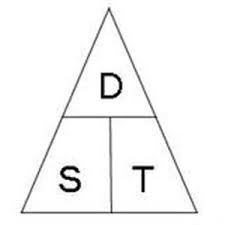• Speed = Total Distance/Total Time, S=D/T
• If D is subject then D=SxT
• If T is subject then T=D/S

Density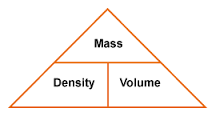• Density = Mass/Volume, D=M/V
• If M is subject then M=DxV
• If V is subject then V=M/D
6 of 28

## Ratios

Dividing an amount into a ratio

• Add up total ratio, i.e £45 split 2:7. Total = 9. Divide amount by ratio total. 45/9 = 5
• Multiply ratios by individual amounts,, 2x5=10 & 7x5=35.
7 of 28

## Indices

Multiplying Indices

If the base number is the same you can then just add the powers

23 × 24 = 27

Dividing Indices

When dividing subtract the powers.

25 ÷ 22 = = 23

Negative Indices

A negative exponent means how many times to divide by the number.

Example: 5-3 = 1 ÷ 5 ÷ 5 ÷ 5 = 0.008

Zero indices = 1

8 of 28

## Indices 2

Fractional Indices

An exponent of 1/2 is actually square root.  eg 9½ × 9½ = 9

An exponent of 1/3 is cube root. 271/3 =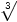27 = 3

An exponent of 1/4 is 4th root

43/2 = 43×(1/2) = √(43) = √(4×4×4) = √(64) = 8

642/3, you have to do cube root of 64 first.  64^1/3 = 4 then square this 4^2 = 16

9 of 28

## Formulae

Changing the subject of a formula

• Add the same variable to both sides.
• Subtract the same variable from both sides.
• Multiply both sides by the same variable.
• Divide both sides by the same variable.
• Square both sides
• Square root both sides.
• Get rid of fractions − multiply throughout by the denominator.
• Break brackets
10 of 28

## Algebraic Functions

Simplyfying Algebraic Functions

• When multiplying fractions, multiply numerator and denominator together
• When adding fractions, use the lowest common denominator and add numerator
• Factorise all expressions fully
• Cancel out like terms top and bottom
• If everything is cancelled replace with a 1• First, factor the numerator and factor the denominator.
• Then divide out the common binomial  factor (+ 2).
11 of 28

## Statistical Measures

Mean Average

Add up all the numbers, then divide by how many numbers there are.

Mode Average

The number which appears most often.

Median Average

The "middle number" (in a sorted list of numbers).

Quartiles

Quartiles are the values that divide a list of numbers into quarters.

Q1 Lower Quartile

Q2 Middle Quartile (median)

Q3 Upper Quartile

Inter-quartile range - The difference between the upper quartile and the lower quartile, from Q1 to Q3
Grouped frequency distribution - shows how often grouped data appears

12 of 28

## HistogramsHistograms groups numbers into ranges. The range of each bar is also called the Class Interval. Histograms are a great way to show results of continuous data, such as weight, height, etc.

13 of 28

## Probability

Mutually exclusive events

Events that cannot happen at the same time

Relative frequency

How often something happens divided by all outcomes.

Example: if your team has won 9 games from a total of 12 games played:

• the Frequency of winning is 9
• the Relative Frequency of winning is 9/12 = 75%
14 of 28

## Independent Events and Tree Diagrams• The probability of getting Sam as a coach is is 0.6, so the probability of Alex must be 0.4
• If you get Sam, there is 0.5 probability of being Goalie (and 0.5 of not being Goalie)
• If you get Alex, there is 0.3 probability of being Goalie (and 0.7 not)
• 0.3 + 0.12 = 0.42 probability of being a Goalkeeper today, that is a 42% chance
15 of 28

## Box and Whisker Plot

Put numbers in order:   3, 4, 4, 4, 7, 10, 11, 12, 14, 16, 17, 18

Cut it into quarters:       3, 4, 4 | 4, 7, 10 | 11, 12, 14 | 16, 17, 18

In this case all the quartiles are between numbers:

• Quartile 1 (Q1) = (4+4)/2 = 4
• Quartile 2 (Q2) = (10+11)/2 = 10.5
• Quartile 3 (Q3) = (14+16)/2 = 15
• The Lowest Value is 3
• The Highest Value is 1816 of 28

## Cumulative frequency diagrams17 of 28

## Transformations

Rotationseg. Rotate triangle 180 degrees at point (-1,1)

ReflectionFlip a shape in a mirror line. e.g. x=3 is a vertical line at x3. Y is a horizontal line

Translation - translating a shape3 right 4up, can use tracing paper, -3 is 3 left. and then -2, is 2 down

18 of 28

## Graph Transformations - Formulas

If the formula is f(x) = x^

If X is -3 then y is  f(-3) = -3^ = 9

-2 then y is f(-2) = -2^ - 4

19 of 28

## Area and circumference of circlesArea = π r 2

Cirmumference = 2 π r

20 of 28

## Angles and Parallel Lines

Vertically Opposite Angles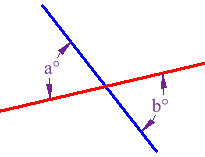Angles opposite each other when two lines cross

TransveralA line that crosses at least two other lines.

Alternate anglesThe pairs of angles on opposite sides of the transversal but outside the two lines are called Alternate Exterior Angles.

21 of 28

## Angles and Parallel Lines II

Corresponding anglesTwo lines are crossed by another line  the angles in matching corners are corresponding angles.

Allied angle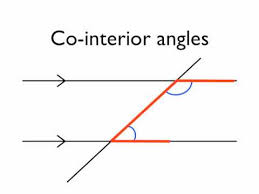is an angle pair, which is created by transversal line.

22 of 28

## Area of Triangles

Multiply the base by the height, and then divide by 2.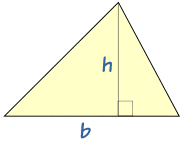23 of 28

## Pythagoras' Theorem

The biggest square has the exact same area as the other two squares put together

a2 + b2 = c2If we know the lengths of two sides of a right angled triangle, we can find the length of the third side

a2 + b2 = c2

If 52 + 122 = c2

25 + 144 = c2

169 = c2

c2 = 169

c = √169

c = 13

24 of 28

## Area of trapeziums

trapezium is a quadrilateral that has only one pair of parallel sides.To find the area of any trapezium, add together the parallel sides, then halve your answer and multiply by the height.

Area = ½ (a + b)h

25 of 28

## Factorising

Expressions

The reverse of expanding brackets.

3p + 6 =  3(p + 2) - 3 is the common factor

4xy - 2x =  2x ( 2y -1 ) - 2 is the common factor

14 - 7d = 7(2 - d) - 7 is the common factorMust add up to the first factor and multiply by second26 of 28

## Solving linear equations

Find x if: 2x + 4 = 10

• Isolate "x" to one side of the equation  by subtracting 4 from both sides
• 2x + 4 - 4 = 10 - 4
• 2x = 6

• Divide both sides by 2
• 2x / 2 = 6 / 2
• x = 3

If any equation has brackets, then deal with the brackets first

27 of 28

## Simultaneous equations

simultaneous equation is two (or more) equations which contain more than one letter term.

• Equation 1: 2x + y = 7
• Equation 2: 3x - y = 8

Add the two equations to eliminate the ys: *subtract if both the same

• 2x + y = 7
• 3x - y = 8
• ------------
• 5x = 15
• x = 3
• Now you can put x = 3 in either of the equations.
• Substitute x = 3 into the equation 2x + y = 7:
• 6 + y = 7
• y = 1

So the answers are x = 3 and y = 1

28 of 28